# Math - Kindergarten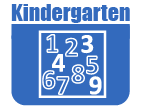Resources by Strand

Strand: MATHEMATICAL PRACTICES (K.MP)
The Standards for Mathematical Practice in Kindergarten describe mathematical habits of mind that teachers should seek to develop in their students. Students will become mathematically proficient in engaging with mathematical content and concepts as they learn, experience, and apply these skills and attitudes (Standards K.MP.18).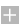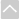Strand: COUNTING AND CARDINALITY (K.CC)
Know number names and the counting sequence (Standards K.CC.1–3).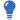Core Guide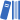OER Curriculum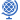Instructional TasksReview Activitiy

Standard K.CC.1.
Count to 100 by ones and by tens.

Standard K.CC.2.
Count forward beginning from a given number within the known sequence (instead of having to begin at 1).

Standard K.CC.3.
Read and write numbers using base ten numerals from 0 to 20. Represent a number of objects with a written numeral, in or out of sequence (0 represents a count of no objects).

Count to tell the number of objects (Standards K.CC. 4–5).Core GuideOER CurriculumInstructional TasksReview Activitiy

Standard K.CC.4.
Understand the relationship between numbers and quantities; connect counting to cardinality.

1. When counting objects, say the numbers in the standard order. Pair each quantity of objects with one and only one number and each number with the correct quantity of objects.
2. Understand that the last number said represents the number of objects counted. The number of objects is the same regardless of their arrangement or the order in which they were counted.
3. Understand that each successive number refers to a quantity that is one greater than the previous number.

Standard K.CC.5.
Use counting to answer questions about "how many." For example, 20 or fewer objects arranged in a line, a rectangular array, or circle; 10 or fewer objects in a scattered configuration. Using a number from 120, count out that many objects.

Identify and compare quantities of objects and numerals (Standards K.CC.6–7).Core GuideOER CurriculumInstructional TasksReview Activitiy

Standard K.CC.6.
Use matching or counting strategies to identify whether the number of objects in one group is greater than, less than, or equal to the number of objects in another group. Include groups with up to ten objects.

Standard K.CC.7
Compare two numbers between 1 and 10 presented as written numerals using "greater than," "less than," or "equal to."Strand: OPERATIONS AND ALGEBRAIC THINKING (K.OA)
Understand addition as putting together and adding to, and understand subtraction as taking apart and taking from (Standards K.OA.1–5).Core GuideOER CurriculumInstructional TasksReview Activitiy

Standard K.OA.1
Represent addition and subtraction with objects, fingers, mental images, simple drawings, or sounds. For example, use clapping, act out situations, and use verbal explanations, expressions, or equations.

Standard K.OA.2
Solve addition and subtraction word problems within 10. Use objects or drawings to represent the problem.

Standard K.OA.3
Decompose numbers less than or equal to 10 into pairs in more than one way by using objects or drawings. Record each decomposition by a drawing or equation. For example, 5 = 2 + 3 and 5 = 4 + 1.

Standard K.OA.4
Make sums of 10 using any number from 1 to 9. For example, 2 + 8 = 10. Use objects or drawings to represent and record the answer.

Standard K.OA.5
Fluently add and subtract using numbers within 5.Strand: NUMBER AND OPERATIONS IN BASE TEN (K.NBT)
Compose and decompose numbers 11–19 to gain foundations for place value (Standard K.NBT.1).Core GuideOER CurriculumInstructional TasksReview Activitiy

Standard K.NBT.1
Compose and decompose numbers from 1119 into ten ones and some further ones. Use objects or drawings and record each composition or decomposition by a drawing or equation. For example, 18 = 10 + 8. Understand that these numbers are composed of ten ones and one, two, three, four, five, six, seven, eight, or nine ones.Strand: MEASUREMENT AND DATA (K.MD)
Describe and compare measurable attributes of objects (Standards K.MD.1–2)Core GuideOER CurriculumInstructional TasksReview Activitiy

Standard K.MD.1
Describe measurable attributes of objects, such as length or weight. Describe several measurable attributes of a single object.

Standard K.MD.2
Directly compare two objects with a measurable attribute in common, to see which object has "more of"/"less of" the attribute, and describe the difference. For example, directly compare the length of two pencils and describe one as shorter or longer.

Classify objects and count the number of objects in each category (Standard K.MD.3).Core GuideOER CurriculumInstructional TasksReview Activitiy

Standard K.MD.3
Classify objects into given categories; count the numbers of objects in each category and sort the categories by count. Limit the category counts to less than or equal to 10.Strand: GEOMETRY (K.G)
Identify and describe shapes, including squares, circles, triangles, rectangles, hexagons, cubes, cones, cylinders, and spheres (Standards K.G.1–3).Core GuideOER CurriculumReview Activitiy

Standard K.G.1
Describe objects in the environment using names of shapes, and describe the relative positions of these objects using terms such as above, below, beside, in front of, behind, and next to.

Standard K.G.2
Correctly name shapes regardless of their orientations or overall sizes.

Standard K.G.3
Identify shapes as two-dimensional ("flat") or three-dimensional ("solid").

Analyze, compare, create, and compose shapes (Standards K.G.4–6).Core GuideOER CurriculumReview Activitiy

Standard K.G.4
Analyze, compare, and sort two- and three-dimensional shapes and objects, in different sizes and orientations, using informal language to describe their similarities, differences, and other attributes (for example, color, size, shape, number of sides).

Standard K.G.5
Model and create shapes from components such as sticks and clay balls.

Standard K.G.6
Compose simple shapes to form larger shapes. For example, "Can you join these two triangles with full sides touching to make a rectangle?"The Online Core Resource pages are a collaborative project between the Utah State Board of Education and the Utah Education Network. If you would like to recommend a high quality resource, contact Trish French (Elementary) or Lindsey Henderson (Secondary). If you find inaccuracies or broken links contact resources@uen.org.